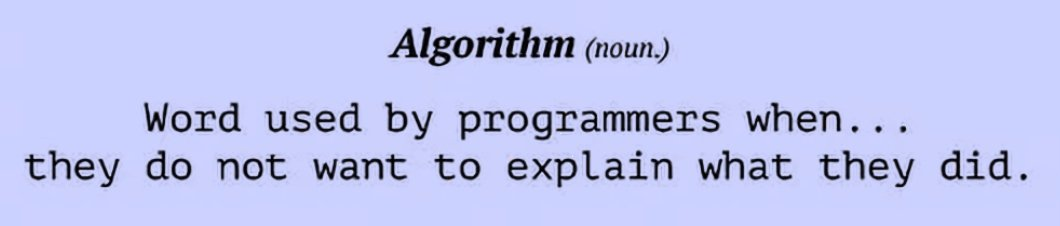Given text documents, we can group them automatically: text clustering. We’ll use KMeans which is an unsupervised machine learning algorithm.

I’ve collected some articles about cats and google. You’ve guessed it: the algorithm will create clusters. The articles can be about anything, the clustering algorithm will create clusters automatically. Even cooler: prediction.

Related course: Complete Machine Learning Course with Python

## Kmeans

We create the documents using a Python list. In our example, documents are simply text strings that fit on the screen. In a real world situation, they may be big files.

## Feature extraction

KMeans normally works with numbers only: we need to have numbers. To get numbers, we do a common step known as feature extraction.

The feature we’ll use is TF-IDF, a numerical statistic. This statistic uses term frequency and inverse document frequency. In short: we use statistics to get to numerical features. Because I’m lazy, We’ll use the existing implementation of the TF-IDF algorithm in sklearn.

The method TfidfVectorizer() implements the TF-IDF algorithm. Briefly, the method TfidfVectorizer converts a collection of raw documents to a matrix of TF-IDF features.## Text clustering

After we have numerical features, we initialize the KMeans algorithm with K=2. If you want to determine K automatically, see the previous article. We’ll then print the top words per cluster.

Then we get to the cool part: we give a new document to the clustering algorithm and let it predict its class. In the code below I’ve done that twice.

If you are new to Machine Learning, I highly recommend this book